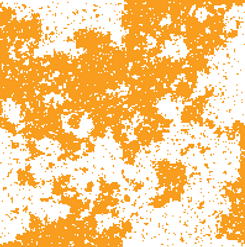I'm a mathematician working on problems motivated by physics, such as conformal field theories (CFT), integrable models, and scaling limits of random planar lattice models.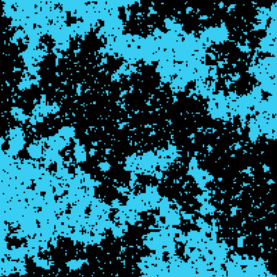In general, I try to combine different mathematical methods to tackle problems related to mathematical physics. My research interests include (but are not limited to) constructive field theory, CFT, correlation functions, crossing probabilities, diagram algebras, Gaussian free field, integrable models, representation theory, Schramm-Loewner evolutions (SLE), quantum groups, and vertex operator algebras. I am particularly interested in understanding algebraic and geometric structures underlying critical random models and CFT.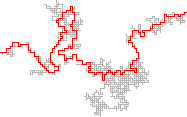Random planar geometry

Making sense of rough objects e.g. using the Gaussian free field and multiplicative chaos. Constructing scaling limits of random planar lattice models.

Schramm-Loewner evolutions

Understanding SLEs and their variants and relating them to critical lattice models and CFT.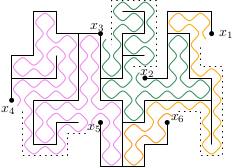Conformal Field Theory

Building rigorous foundations for 2D CFT. Making sense of CFTs as scaling limits of critical lattice models. Using tools from CFT in probabilistic problems.

Algebraic structures

Temperley-Lieb algebra, its variants, and quantum groups in relation with critical models, SLE, CFT.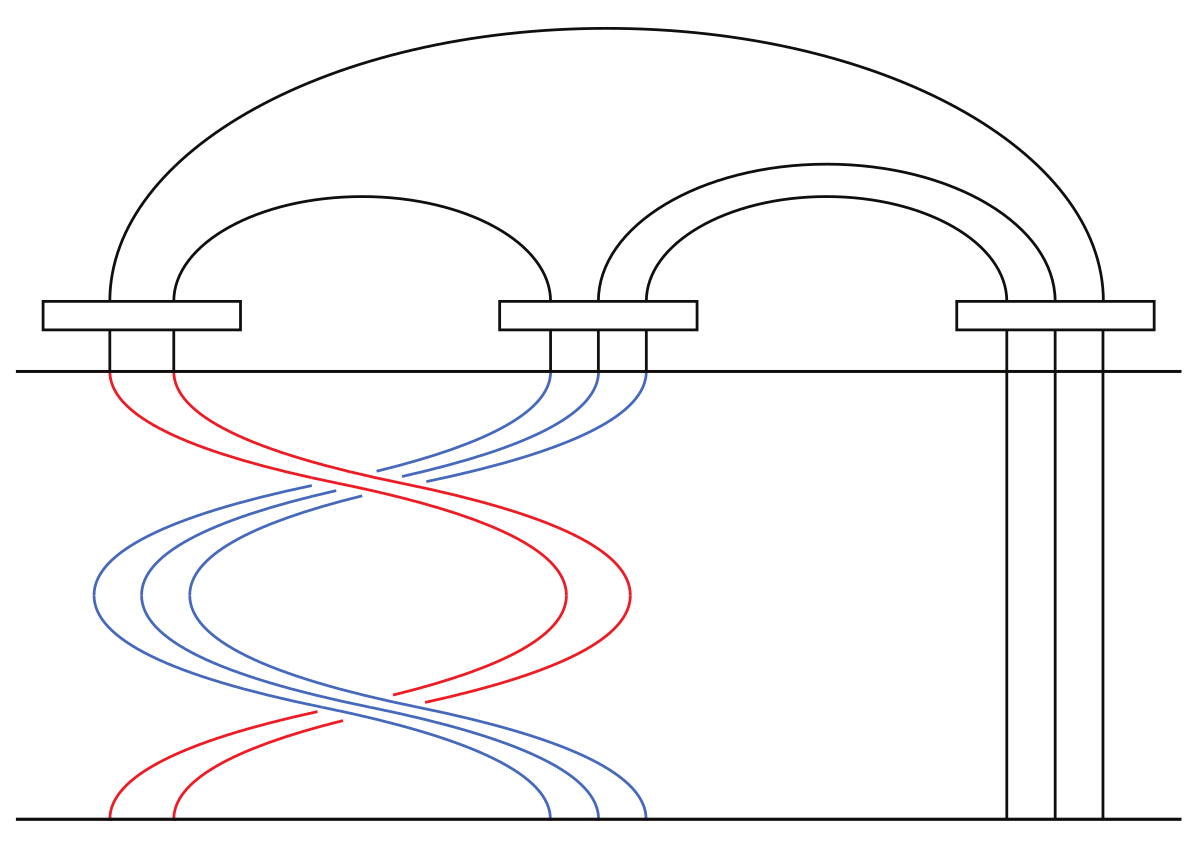Critical phenomena and integrability

Understanding statistical physics models at and near criticality. Finding exact solutions and formulas.

Biography:

Currently I work at Aalto Uni (Finland) and at Uni Bonn (Germany).

Brief job history and current activities:

I did my studies & PhD (2016) at University of Helsinki, where I mostly studied mathematics (major) and theoretical physics (minor), with a glimpse of other sciences.

Education: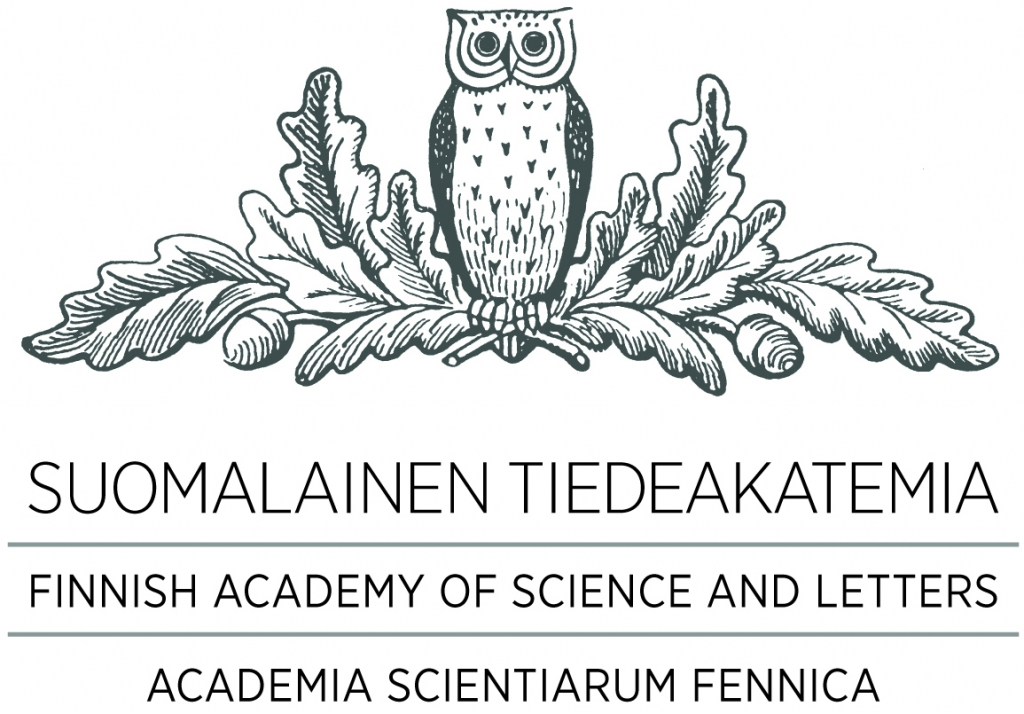University of Helsinki

Department of Mathematics and Statistics

09/2016: Doctor of Philosophy (Mathematics)

02/2012: Master of Science (Mathematics)

12/2010: Bachelor of Science (Mathematics)

During 2012-2016, my graduate research was supported by the Vilho, Yrjö and Kalle Väisälä Foundation (Finnish Academy of Science and Letters), and by the Finnish National Doctoral Programme in Mathematics and its Applications (University of Helsinki).